# Graph Theory (Advance Level) - 1

## 12 Questions MCQ Test Question Bank for GATE Computer Science Engineering | Graph Theory (Advance Level) - 1

Description
This mock test of Graph Theory (Advance Level) - 1 for GATE helps you for every GATE entrance exam. This contains 12 Multiple Choice Questions for GATE Graph Theory (Advance Level) - 1 (mcq) to study with solutions a complete question bank. The solved questions answers in this Graph Theory (Advance Level) - 1 quiz give you a good mix of easy questions and tough questions. GATE students definitely take this Graph Theory (Advance Level) - 1 exercise for a better result in the exam. You can find other Graph Theory (Advance Level) - 1 extra questions, long questions & short questions for GATE on EduRev as well by searching above.
QUESTION: 1

### Let G be an arbitrary graph with n nodes and k components. If a vertex is removed from G, the number of components in the resultant graph must necessarily lie between.

Solution:

Maximum components will result after removal of a node if graph G is a star graph as shown below.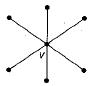or a null graph of n vertices as shown below: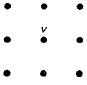In either case, if node vis removed, the number of components will be n - 1, where, n is the total number of nodes in the star graph.
∴ n - 1 is the maximum number of components possible. Minimum components will result if the node being removed is a lone vertex in which case, the number of components will be k - 1.
∴ The number of components must necessarily lie between k - 1 and n - 1.

QUESTION: 2

### How many perfect matchings are there in a complete graph of 6 vertices?

Solution:

The number of perfect matchings in a complete graph of n vertices, where n is even, reduces to the problem of finding unordered partitions of the vertex set of the type p(2n; 2, 2, 2, ... n times)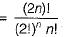For n = 3, 2n = 6, i.e. complete graph K6, we have
Number of perfect matchings =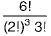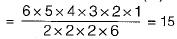QUESTION: 3

### A Graph G = (V, E) satisfies |E| ≤ 3 |V| - 6. The min-degree of G is defined as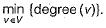Therefore, min-degree of G cannot be

Solution: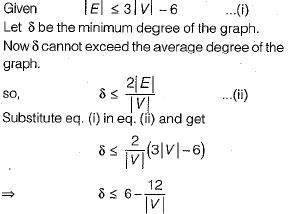Clearly the minimum degree has to be less than 6 always and hence cannot be equal to 6.

QUESTION: 4

What is the number of vertices in an undirected connected graph with 27 edges, 6 vertices of degree 2, 3 vertices of degree 4 and remaining of degree 3?

Solution:

Sum of degree of all vertices = 2e (using Handshaking lemma)
So, 6 x 2 + 3 x 4 + x x 3 = 2 x 27
12 + 12 + 3x = 54
3x = 54 - 24
x = 30/3
x = 10
So, total number of vertices
⇒ x + 6 + 3 = 10 + 6 + 3 = 19

QUESTION: 5

The number of connected components in Gis

Solution:

The number of connected component of G is determined by the degree and edges of vertices there are n + 1 vertices whose degree is zero, so they can form n + 1 connected component. The remaining vertices of the graph Gare all connected as a single component. So total number of connected component is n + 2.

QUESTION: 6

Which of the following graphs has an Eulerian circuit?

Solution:

Whenever in a graph all vertices have even degrees, it will surely have an Euler circuit.
(a) Since in a k-regular graph, every vertex has exactly k degrees and if k is even, every vertex in the graph has even degrees, kregular graph need not be connected, hence k-regular may not contain Euler circuit.
(b) Complete graph on 90 vertices not contains an Euler circuit, because every vertex degree is odd (89).
(c) C25 has 24 edges and each vertex has exactly 2 degrees. So every vertex in the complement of C25 will have 24 - 2 = 22 degrees which is an even number.
Since every vertex in the complement of C25 has even degrees. Also, the complements of all Cn with n ≥ 5 is connected. So, it is an Euler graph.

QUESTION: 7

G is a simple, connected, undirected graph. Some vertices of G are of odd degree. Add a node v to G and make it adjacent to each odd degree vertex of G. The resultant graph is sure to be

Solution:

In an undirected simple, connected graph number of vertices must be even of odd degree (using Handshaking lemma).
Adding a vertex v, adjacent to all odd degree vertices in graph, so degree of all odd degree vertices now become even and degree of vertex v is also even (since number of odd degree vertex are even).
Now all vertices in the graph are of even degree and graph is connected, so it must be Eular graph.

QUESTION: 8

The degree sequence of a simple graph is the sequence of the degrees of the nodes in the graph in decreasing order. Which of the following sequences can not be the degree sequence of any graph?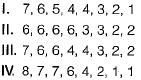Solution:

Havell-Hakimi algorithm can be used to check whether a given degree sequence is a graph or not.
The algorithm is
1. Remove top node of the sequence.
2. Subtract “1 ” from as many nodes in remaining sequence as the degree of top node that was removed.
3. Rearrange this sequence in non increasing order.
4. Check if resulting sequence is a graph.
5. Proceed again to step 1.
If the given sequence is not a graph we will see a violation in step 4, such as presence of negative degrees in the sequence. Otherwise the algorithm will bottom out with a degree sequence consisting of only even number of 1’s and any number of 0’s.
Now applying the algorithm to the degree sequences I, II, III and IV, one by one:
1.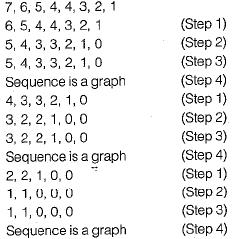Now, the algorithm ends, since the sequence has only 0’s and even number of 1’s.
The final sequence corresponds to following valid graph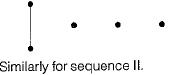2.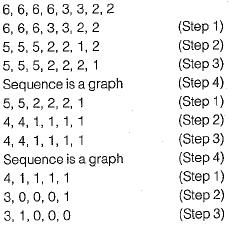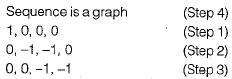The sequence is not a graph (Step 4), since negative degrees not possible in a valid graph. So, algorithm ends.
II is cannot be the degree sequence of any.graph. Similarly we can show that III is degree sequence of some graph and IV is not a degree sequence of any graph.

QUESTION: 9

Which of the following graphs is isomorphic to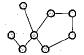Solution:

Check invariants are one by one.
Step 1: All 4 choice have same number of vertices and edges as given graph.
Step 2: So we find degree sequence of given graph which is (1,1, 2, 2, 2, 2, 2, 4).
Degree sequence of graph in option (a) is (1, 1, 1, 2, 2, 2, 3, 4).
Degree sequence of graph in option (b) is (1, 1, 2, 2, 2, 2, 2, 4)
Degree sequence of graph in option (c) is (1, 1,2, 2, 2, 2, 3, 3)
Degree sequence of graph in option (d) is ( 1 , 1 , 2, 2, 2, 2, 2 , 4 ) .
So only options (a) and (c) are not isomorphic to given graph, since degree sequence of these graphs is not same as given graph.
Step 3: Now to decide between options (b) and (d), which one is isomorphic to given graph, we check the number of cycles.
In given Graph there is one cycle of length 5 but in Graph (d), there is no cycle of length 5.
Graph (b) has one cycle of length 5.
So only Graph (b) can be isomorphic to given Graph.

QUESTION: 10

Which of the following statements is/are TRUE for undirected graph?
P: Number of odd degree vertices is even.
Q: Sum of degrees of all vertices is even.

Solution:

Q is Hand-shaking theorem and hence true.
P is a corollory to the Hand-shaking theorem and hence also true.

QUESTION: 11

An ordered n-tuple (d1, d2, .... dn) with d1 ≥ d≥ ... ≥ dn is called graphic if there exists a simple undirected graph with n vertices having degrees d1, d2, ..., dn respectively. Which of the following 6-tuples is NOT graphic?

Solution:

Use the Havell-hakimi algorithm.
The sequence of steps in the algorithm for each graph is shown below: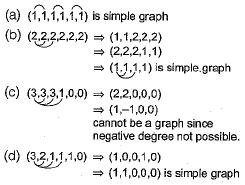QUESTION: 12

If G is a forest with n-vertices and k connected components, how many edges does G have?

Solution:

The number of edges in spanning forest of a graph G with n vertices and k components = Rank (G) = n - k .